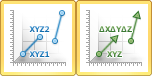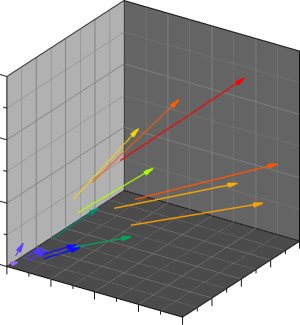# XYZ Vector Plots

 Click the Home | New Graph | Vector | XYZ 2-Point Vector Plot or Home | New Graph | Vector | XYZ-dx,dy,dz Vector Plot command to create an XYZ vector plot. Vector plots create vector lines between two points or from one point to the X+dx, Y+dy, and Z+dz values. When symbols are displayed on 3D vector plots, symbols are shown at the beginning of the vector line. When arrows are displayed on a 3D vector plot, the arrows are shown at the end of the vector line. Click the XYZ 2-Point Vector Plot or XYZ-dx,dy,dz Vector Plot button to create a vector plot.XYZ 2-Point Vector Plots are created from six data columns. Three columns specify the starting X, Y, and Z location. Three other columns specify the ending X, Y, and Z location. A straight line with a vector arrow is drawn between the two points.

XYZ-dx,dy,dz Vector Plots are also created from six data columns. Three columns specify the starting X, Y, and Z location. Three other columns specify the magnitude in the X, Y, and Z direction (dx, dy, and dz) for the vector. A straight line is drawn from the starting X, Y, Z location to the X+dx, Y+dy, Z+dz location.Vector plots show data as
straight-line segments.

## Creating a New XYZ Vector Plot

To create an XYZ vector plot:

1. Click the Home | New Graph | Vector | XYZ 2-Point Vector Plot or Home | New Graph | Vector | XYZ-dx,dy,dz Vector Plot command.
2. Select a worksheet file in the Open Worksheet dialog. You can select a new data file or you can select an open worksheet file in the Open worksheets section.
3. Click the Open button. A 3D vector plot is created using the default properties.

## Editing 3D Vector Plot Properties

To change the features of the vector plot, including the columns used to create the plot, first select the plot in the plot window or Object Manager and then edit its properties in the Property Manager.

Click the following tabs in the Property Manager to change different properties: